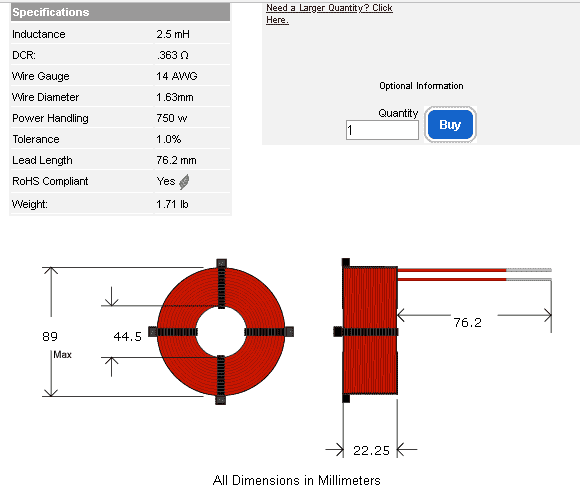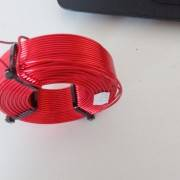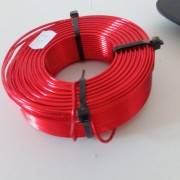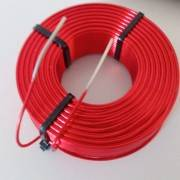# How do I find out the flux for an air core inductor

Mentor
How do I find out gauss from air core inductor 36mohm pumped with ~130amps 9ms long?

http://www.parts-express.com/erse-25mh-14-awg-perfect-layer-inductor-crossover-coil--266-380

Greatly appriciated.
Welcome to the PF.

The coil resistance and the pulse time don't matter for the initial calculation of the core flux. What have you found in your initial searching for the core flux of an air core solenoid based on the Amp-Turns? What is the application, and what is your background? How are you generating that 130 Amps?

pager48
Welcome to the PF.

The coil resistance and the pulse time don't matter for the initial calculation of the core flux. What have you found in your initial searching for the core flux of an air core solenoid based on the Amp-Turns? What is the application, and what is your background? How are you generating that 130 Amps?

Current is dumped from charged 4.7mF capacitor, I can however use 4 12v batteries to achieve the same current and magnetism. Application requires ~6k gauss field, the only reason I am inquiring about this is because I do NOT have the turn count, if I did then its a simple geometric calculation. In fact I should just use the batteries and not bother with rectifying mains power to charge a cap. I can probably get a higher duty cycle out of batteries.

Gold Member
Just looking at the photos, I count 16 layers and either 13 or 14 turns per layer. That yields 208 to 224 turns. That should give you a starting point.

For a sanity check 1; 14AWG copper wire is 80.44 feet per pound and the data sheet says 2.244 pounds, or 180 feet of wire.

For sanity check 2; data sheet shows 3.69 inches O.D. Take the average of the I.D. and the O.D. then multipy by pi for the average circumference. Divide 180 feet by the average circumference to get the number of turns.

For sanity check 3; Resistance of 14AWG is 396 feet per Ohm, times by 0.36 gives 142 feet of wire.

Using the above and 216 turns, predicts the I.D is (very) roughly 2-7/8 inches.

Does that match?

Last edited:
•dlgoff, berkeman and jim hardy
Gold Member
Dearly Missed
Late Edit - oops- i see @Tom.G posted while i was typing. I don't think we disagree on the basics, though.

I am inquiring about this is because I do NOT have the turn count, if I did then its a simple geometric calculation.
Do you have a coil in hand? Seems easy enough to count the layers and get pretty close.

Here's the dimensions of your coil from Erse's webpage
#14 wire is 1.63 mm diameter which puts upper limits on number of layers and number of turns per layer.
I get 22.25/1.63 = 13 layers max, 13 turns max per layer = 169 turns maximum.
A customer comment on the Parts-Express page suggests about 135 turns, 80% of calculated maximum.Calculating flux around a flat coil is not easy because the flux isn't uniform. It spreads out rapidly from the ends.

You do understand Gauss is flux density, right ?

Where do you want that 6000 Gauss? Center of coil or at an end? A foot away ?

I'd make a search coil of known area and place it where you want to produce that 6000 Gauss.
Then put one amp of 60 hz current through the inductor .

Since e = n X dΦ/dt X 10^-8 and Φ is flux in Maxwells not flux density in Gauss
Voltage induced in your search coil will be 120pi X (search coil area in mm2) X (Gauss) X 10^-10 volts per turn.
(check my algebra)
So you'll know Gauss per amp at that particular spot.

If you make a search coil of say ten turns on 44.5 mm diameter, same as the inductor's center hole,
then at 60 hz , 6000 Gauss rms through it would give 3.518 volts rms. (check my arithmetic)
17 turns instead would give very nearly 1 volt per kiloGauss which might be handy.

But - at one amp you'll get nowhere near 6 kiloGauss or even 1 kiloGauss.
2.5 milliHenries at 60 hz is 0.942 ohms , so with an amp through it voltage across the entire inductor will be ~ 0.942 volts due to flux (and 0.353 volts due to resistance..)
If the inductor has 135 turns that's only about 7 millivots per turn from flux, suggesting more like 120 Gauss.
That's good reason to make a 17 turn search coil instead of a single turn. With 17 turns you should find about 0.12 volts per ampere right adjacent the inductor's end.
And you can measure how fast it drops off as you move away.

You should be able to duplicate my logic and arithmetic. Keep us posted on your experiments ?

What are you doing - recharging magneto magnets?

old jim

#### Attachments

Last edited:
•dlgoff and berkeman
pager48
So I just recently received the inductor and as you can see it came a bit banged up infact it was banged up so hard that one zip tie borke off, but the company is shipping a new one for absolutely free and told me to keep the one I have. What I don't understand is which inductor a donut shaped inductor like the one I have or an inductor which has no empty center (not donut) radiate a magnetic field further supposing same inductance & power pumped into each coil?